﻿ C : Delete node from the beginning of a doubly linked list

# C Exercises: Delete node from the beginning of a doubly linked list

## C Doubly Linked List : Exercise-7 with Solution

Write a program in C to delete a node from the beginning of a doubly linked list.

Pictorial Presentation: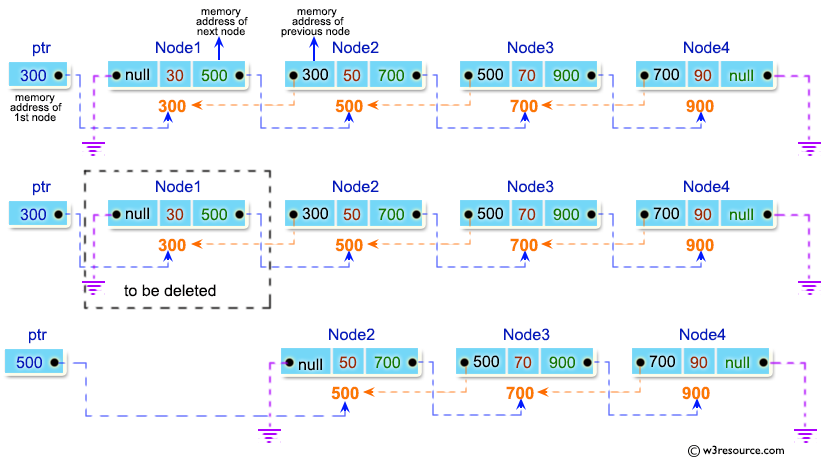Sample Solution:

C Code:

``````#include <stdio.h>
#include <stdlib.h>

struct node {
int num;
struct node * preptr;
struct node * nextptr;
}*stnode, *ennode;

void DlListcreation(int n);
void DlListDeleteFirstNode();
void displayDlList(int a);

int main()
{
int n,num1,a,insPlc;
stnode = NULL;
ennode = NULL;
printf("\n\n Doubly Linked List : Delete node from the beginning of a doubly linked list :\n");
printf("----------------------------------------------------------------------------------\n");
printf(" Input the number of nodes (3 or more ): ");
scanf("%d", &n);
DlListcreation(n);
a=1;
displayDlList(a);
DlListDeleteFirstNode();
a=2;
displayDlList(a);
return 0;
}

void DlListcreation(int n)
{
int i, num;
struct node *fnNode;

if(n >= 1)
{
stnode = (struct node *)malloc(sizeof(struct node));
if(stnode != NULL)
{
printf(" Input data for node 1 : "); // assigning data in the first node
scanf("%d", &num);
stnode->num = num;
stnode->preptr = NULL;
stnode->nextptr = NULL;
ennode = stnode;
for(i=2; i<=n; i++)
{
fnNode = (struct node *)malloc(sizeof(struct node));
if(fnNode != NULL)
{
printf(" Input data for node %d : ", i);
scanf("%d", &num);
fnNode->num = num;
fnNode->preptr = ennode;    // new node is linking with the previous node
fnNode->nextptr = NULL;     // set next address of fnnode is NULL
ennode->nextptr = fnNode;   // previous node is linking with the new node
ennode = fnNode;            // assign new node as last node
}
else
{
printf(" Memory can not be allocated.");
break;
}
}
}
else
{
printf(" Memory can not be allocated.");
}
}
}

void DlListDeleteFirstNode()
{
struct node * NodeToDel;
if(stnode == NULL)
{
printf(" Delete is not possible. No data in the list.\n");
}
else
{
NodeToDel = stnode;
stnode = stnode->nextptr;   // move the next address of starting node to 2 node
stnode->preptr = NULL;      // set previous address of staring node is NULL
free(NodeToDel);            // delete the first node from memory
}
}

void displayDlList(int m)
{
struct node * tmp;
int n = 1;
if(stnode == NULL)
{
printf(" No data found in the List yet.");
}
else
{
tmp = stnode;
if (m==1)
{
printf("\n Data entered in the list are :\n");
}
else
{
printf("\n After deletion the new list are :\n");
}
while(tmp != NULL)
{
printf(" node %d : %d\n", n, tmp->num);
n++;
tmp = tmp->nextptr; // current pointer moves to the next node
}
}
}
```
```

Sample Output:

``` Doubly Linked List : Delete node from the beginning of a doubly linked list :
----------------------------------------------------------------------------------
Input the number of nodes (3 or more ): 3
Input data for node 1 : 1
Input data for node 2 : 2
Input data for node 3 : 3

Data entered in the list are :
node 1 : 1
node 2 : 2
node 3 : 3

After deletion the new list are :
node 1 : 2
node 2 : 3
```

Flowchart: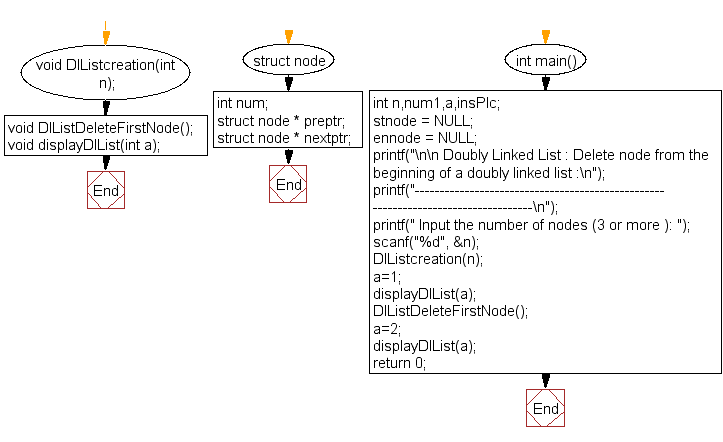DlListcreation() :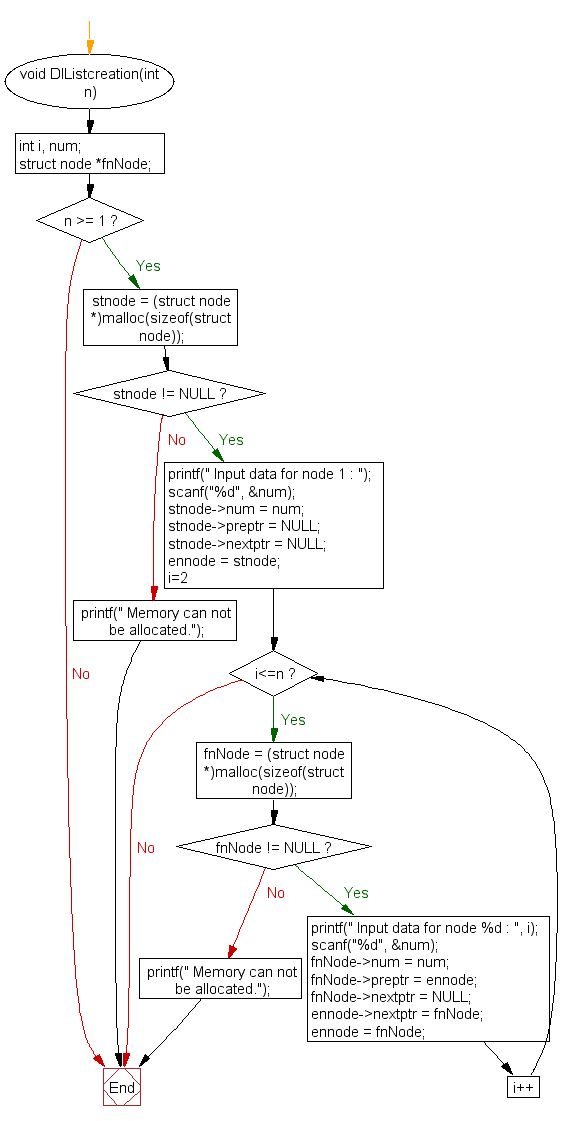DlListDeleteFirstNode() :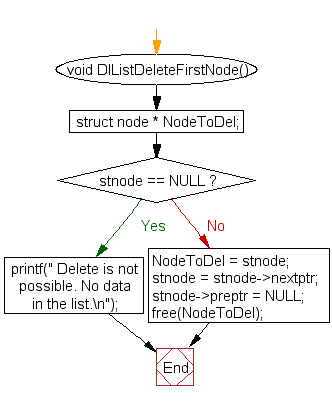displayDlList() :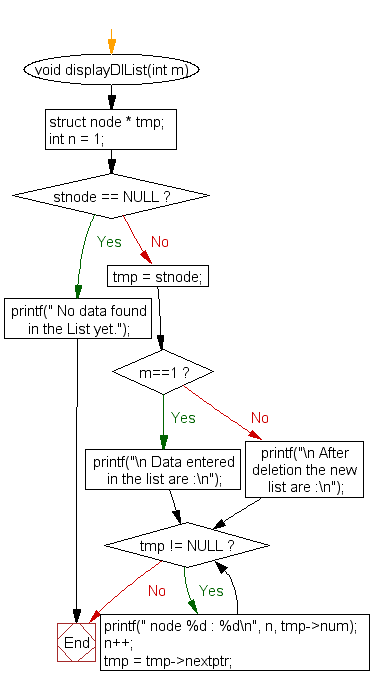C Programming Code Editor:

What is the difficulty level of this exercise?

Test your Programming skills with w3resource's quiz.

﻿